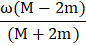# Two particles of massesandand velocitiesandmake an elastic head on collision. If the initial kinetic energies of the two particles are equal andcomes to rest after collision, then a)b)c)d)## Question ID - 100779 :- Two particles of massesandand velocitiesandmake an elastic head on collision. If the initial kinetic energies of the two particles are equal andcomes to rest after collision, then a)b)c)d)3537

(a,c)

Since both masses have equal kinetic energy, so(i)

By law of conservation of momentum(ii)

Further by law of conservation of energy we have(iii)

From Eqs. (ii) and (iii), we getNext Question :
 A thin circular ring of massand radiusis rotating about its axis with a constant angular velocity. Two objects, each of mass, are attached gently to the opposite ends of the diameter of the ring. The wheel now rotates with an angular velocity a)b)c)d)